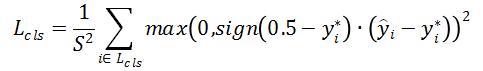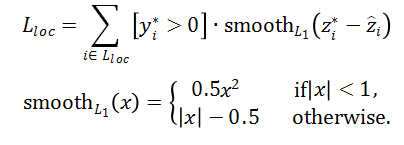# Deep Direct Regression for Multi-Oriented Scene Text Detection

On the ICDAR2015 Incidental Scene Text benchmark, proposed method achieves F1-measure of 81%.

## 1. Introduction

Text detection is a procedure that determines whether the text is present in natural images and, if it is, where each text instance is located. Text in images provides rich and precise high-level semantic information, which is important for numerous potential applications, such as scene understanding, image and video retrieval, and content-based recommendation systems. Consequently, scene text detection has drawn great interests from both computer vision and machine learning communities. In recent years, deep convolutional neural network (CNN) based methods for generic object detection, like Faster-RCNN, SSD, and YOLO, have been proposed and can reach the state-of-the-art performance. Based on these methods, scene text detection has also been greatly improved by regarding text words or lines as objects. However, for multi-oriented text detection, methods like Faster-RCNN and SSD which work well for the object and horizontal text detection may not be good choices.

The authors first provide a new perspective to divide existing high-performance object detection methods into direct and indirect regressions. Direct regression performs boundary regression by predicting the offsets from a given point (see Fig.1.b), while indirect regression predicts the offsets from some bounding box proposals (see Fig.1.a). Then, they analyze the drawbacks of the indirect regression, followed by the recent state-of-the-art detection structures like Faster-RCNN and SSD for multi-oriented scene text detection, and pointed out the potential superiority of direct regression. To verify this point of view, a deep direct regression-based method for multi-oriented scene text detection was proposed. The detection framework is simple and effective, with a fully convolutional network and one-step post-processing. The fully convolutional network is optimized in the end-to-end fashion and has bi-task outputs where one is pixel-wise classification between text and non-text, and the other is direct regression to determine the vertex coordinates of quadrilateral text boundaries. The proposed method is particularly beneficial for localizing incidental scene texts. On the ICDAR2015 Incidental Scene Text benchmark, the proposed method achieves the F1-measure of 81%. On other standard datasets with focused scene texts, the method also reaches good performance.Figure 1 – Visualized explanation of indirect and direct regression.(a) The indirect regression predicts the offsets from a proposal. (b) The direct regression predicts the offsets from a point.

## 2. Theoretical Perspective

The diagram of the proposed detection system is shown in Fig.2 below. It consists of four major parts: the first three modules as the network parts(convolution feature extraction, multi-level feature fusion, and multi-task learning), and an improved NMS (Non-Maximum Suppression) algorithm doing post-processing.

The complete network structure of the method is shown in Figure 3. Given the input image of size m*n, it goes through the convolution feature extraction and downsampling process, and then the three feature fusion process (similar to Resnet’s approach). After each fusion, the deconvolution operation is done. Finally, we obtain the m/4*n/4*128 output features.

The next part is multitask learning, which consists of two sub-tasks: classification task and regression task. On one hand, the output created by the above process is subsequently fed into the classification module. The output of the classification task M_cls is a m/4*n/4 two-order tensor. The element in the tensor represents a score. The higher the score, the more likely that this position is the text, otherwise it is non-text. The output feature, on the other hand, is the input of the regression module, and the output of the regression task M_loc is a m/4*n/4*8 third-order tensor. The channel size indicates that we need to get the coordinates of the four vertices of the text boundary quadrilateral. The value of the third order tensor M_loc in the index (w,h,c) is expressed as M_(w,h,c), representing the offset of the quadrilateral vertex coordinates to the midpoint of the input image(4w,4h). Thus, the text boundary quadrilateral coordinates obtained in the regression task, when being mapped to the original input image, needs to be expanded four times and can be expressed as B(w,h):By combining classification and regression tasks, the network model can predict the quadrilateral coordinates and classification scores for each point in the feature graph m/4*n/4. The details of the network structure and parameter configuration are shown in Figure 3.

### Loss Function

The multi-task loss function L of the network can be represented aswhere, L_cls and L_loc represent the loss for classification task and the regression task, respectively, and the balance between the two losses is controlled by the super parameters ρ_loc

In the classification task, for the selection of the Ground Truth, the authors don’t use all the pixels in the text area as the pixels of the positive sample, but rather the pixels that are less than a certain distance from the centerline of the text. The authors set the distance r as 0.2, and it is proportional the short side of the text boundary. In addition, the short side of the text boundary of the positive sample is limited to [32*2^-1,32*2^1] . That’s to say, if the short side range is within [32*2^-1.5,32*2^-1) ∪ (32*2^1,32*2^1.5], called NOT CARE area, then the text is a negative sample. Around the positive sample, pixels will see the NOT CARE area as the transition boundary between a positive sample and a negative sample. NOT CARE does not participate in the training process. The author argues that this ground truth design can make the boundaries between the text area and the non-text area clearer.

The loss function used for the classification task is Hinge Loss, as follows: where sign (x) is a sign function, and when y^and y* are equal (for example, 1 or 0), then the square is 0 – that is, there is no loss; if not equal, then the square result is 1.In the regression task, the ground truth is distributed within a larger range of values, and the output of the sigmoid layer of the network is within the range {0,1}, so the Scale & Shift module is added to the network. The output of the network is therefore controlled between the range [-400, 400], and the function of the module isAccording to , the loss function \$L_loc\$ in the regression task is defined as follows. For a given pixel, its true value is expressed as z* , and its predicted value is expressed as z^.Non-Maximum Suppression

After multitask learning (classification and regression), each point of the output feature vector (m/4*n/4) corresponds to a quadrilateral box. The output feature vector of the classification task preserves the score of each quadrilateral box. The output feature vector of the regression task, on the other hand, preserves the offset of the coordinates of the four points of each quadrilateral box. In order to filter out some non-text areas, the authors only keep points that score higher after the classification. But even so, there are still some dense overlapping quadrilaterals, which needs to be removed using Recalled NMS.

Recalled NMS is divided into three steps: the first step is to use the traditional NMS algorithm to get the results. The main problem of the results is that when two texts are very close to each other, there are some frames across the two text, which is incorrect. In the second step, each text box obtained from the first step is switched to the text box which has not been processed by NMS, and with the highest score and larger than some threshold. The third step is to merge the box obtained in the second step, because the overlap between the text box at this time is relatively high, and the boxes obtained in the first step – the NMS process – with higher overlap rate have already been removed.

The three steps are shown in Figure 4.

## 3. Experiments

The proposed method was evaluated on three datasets: ICDAR2015 Incidental Scene Text, MSRA-TD500, and ICDAR2013. Those three datasets contain training images, test images, and annotations. The ICDAR2015 dataset contains texts with various scales, resolution, blurring, orientations, and viewpoint, while texts in the ICDAR2013 are well captured in high resolution and clear. Besides, the ICDAR2015 and MSRA-TD500 dataset have multi-oriented texts and the third one has mostly horizontal texts.

Standard evaluation protocols which are provided by the dataset creators or competition organizers are followed in this experiment. The three evaluation metrics are precision, recall, and f-measure. In general, the greater the value of these metrics, the better the experimental algorithm.

The network is optimized by stochastic gradient descent (SGD) with back-propagation, trained on training datasets from ICDAR2013 and ICDAR2015, as well as 200 negative images (scene images without text) collected from the Internet.
Experimental results are shown in following tables. The results shown in Tab.1 demonstrates that the proposed method outperforms previous approaches by a large margin in both precision and recall. However, evaluation results on MSRA-TD500 dataset and ICDAR2013 Focused Scene Text dataset shown in Tab.2 and Tab.3 indicated that the proposed method does not have much advantage. We can make a conclusion that the proposed method really works well for multi-oriented text detection, as the ICDAR2015 dataset has mostly multi-oriented texts. In addition, apart from the precision, recall, and F-measure, the time cost of a method for per image is listed in Tab.3.

## 4. Conclusion

In this paper, the authors first partition existing object detection frameworks into direct and indirect regression-based methods and analyze the pros and cons of both methods for irregularly shaped object detection. Then, they proposed a novel direct regression-based method for multi-oriented scene text detection. The detection framework is straightforward and effective with only one-step post-processing. Moreover, it performs particularly well for incidental text detection. On the ICDAR2015 Incidental Scene Text benchmark, the proposed method has achieved good performance. Apart from this, this paper also analyzes the reasons of the high performance and compare the proposed method to other recent scene text detection systems.

## Reference

D. Erhan, C. Szegedy, A. Toshev, and D. Anguelov. Scalable object detection using deep neural networks. In Proceedings of the IEEE Conference on Computer Vision and Pattern Recognition, 2014. 1, 3
H.Chen,S.S.Tsai,G.Schroth,D.M.Chen,R.Grzeszczuk, and B. Girod. Robust text detection in natural images with edge-enhanced maximally stable extremal regions. In Proceedings of the 18th IEEE International Conference on Image Processing, pages 2609–2612. IEEE, 2011. 2
R. Girshick. Fast r-CNN. In Proceedings of the IEEE International Conference on Computer Vision, pages 1440–1448, 2015. 1, 3, 4

Author: Kejin Jin  | Technial reviewer: Haojin Yang
Paper Source: https://arxiv.org/pdf/1703.08289.pdf
Paper authors: Wenhao He, Xu-Yao Zhang, Fei Yin, Cheng-Lin Liu
National Laboratory of Pattern Recognition (NLPR)
Institute of Automation, Chinese Academy of Sciences, Beijing, China1.